# SHMS Intermediate MathCollection Resources(644)

View
Selected filters:
• Middle School
• Mathematics
• EngageNY
• Illuminations
• Illustrative Mathematics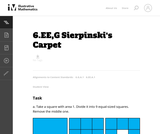Conditions of Use:
Remix and Share
Rating

This is a task from the Illustrative Mathematics website that is one ...

This is a task from the Illustrative Mathematics website that is one part of a complete illustration of the standard to which it is aligned. Each task has at least one solution and some commentary that addresses important asects of the task and its potential use. Here are the first few lines of the commentary for this task: Take a square with area 1. Divide it into 9 equal-sized squares. Remove the middle one. What is the area of the figure now? Take the remaining 8 square...

Subject:
Mathematics
Material Type:
Activity/Lab
Provider:
Illustrative Mathematics
Provider Set:
Illustrative Mathematics
Author:
Illustrative Mathematics
Date created
11-محرم-1436Conditions of Use:
Remix and Share
Rating

This is a task from the Illustrative Mathematics website that is one ...

This is a task from the Illustrative Mathematics website that is one part of a complete illustration of the standard to which it is aligned. Each task has at least one solution and some commentary that addresses important asects of the task and its potential use. Here are the first few lines of the commentary for this task: A penny is about $\frac{1}{16}$ of an inch thick. In 2011 there were approximately 5 billion pennies minted. If all of these pennies were placed in a s...

Subject:
Mathematics
Material Type:
Activity/Lab
Provider:
Illustrative Mathematics
Provider Set:
Illustrative Mathematics
Author:
Illustrative Mathematics
Date created
11-محرم-1436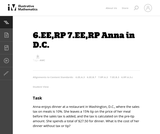Conditions of Use:
Remix and Share
Rating

This is a task from the Illustrative Mathematics website that is one ...

This is a task from the Illustrative Mathematics website that is one part of a complete illustration of the standard to which it is aligned. Each task has at least one solution and some commentary that addresses important asects of the task and its potential use. Here are the first few lines of the commentary for this task: Anna enjoys dinner at a restaurant in Washington, D.C., where the sales tax on meals is 10%. She leaves a 15% tip on the price of her meal before the s...

Subject:
Mathematics
Material Type:
Activity/Lab
Provider:
Illustrative Mathematics
Provider Set:
Illustrative Mathematics
Author:
Illustrative Mathematics
Date created
11-محرم-1436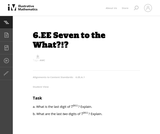Conditions of Use:
Remix and Share
Rating

This is a task from the Illustrative Mathematics website that is one ...

This is a task from the Illustrative Mathematics website that is one part of a complete illustration of the standard to which it is aligned. Each task has at least one solution and some commentary that addresses important asects of the task and its potential use. Here are the first few lines of the commentary for this task: What is the last digit of $7^{2011}$? Explain. What are the last two digits of $7^{2011}$? Explain....

Subject:
Mathematics
Material Type:
Activity/Lab
Provider:
Illustrative Mathematics
Provider Set:
Illustrative Mathematics
Author:
Illustrative Mathematics
Date created
11-محرم-1436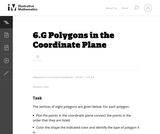Conditions of Use:
Remix and Share
Rating

This is a task from the Illustrative Mathematics website that is one ...

This is a task from the Illustrative Mathematics website that is one part of a complete illustration of the standard to which it is aligned. Each task has at least one solution and some commentary that addresses important asects of the task and its potential use. Here are the first few lines of the commentary for this task: The vertices of eight polygons are given below. For each polygon: * Plot the points in the coordinate plane connect the points in the order that they a...

Subject:
Mathematics
Material Type:
Activity/Lab
Provider:
Illustrative Mathematics
Provider Set:
Illustrative Mathematics
Author:
Illustrative Mathematics
Date created
11-محرم-1436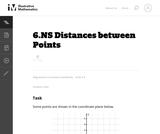Conditions of Use:
Remix and Share
Rating

This is a task from the Illustrative Mathematics website that is one ...

This is a task from the Illustrative Mathematics website that is one part of a complete illustration of the standard to which it is aligned. Each task has at least one solution and some commentary that addresses important asects of the task and its potential use. Here are the first few lines of the commentary for this task: Some points are shown in the coordinate plane below. What is the distance between points B & C? What is the distance between points D & B? What is the ...

Subject:
Mathematics
Material Type:
Activity/Lab
Provider:
Illustrative Mathematics
Provider Set:
Illustrative Mathematics
Author:
Illustrative Mathematics
Date created
11-محرم-1436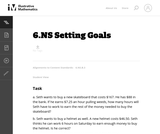Conditions of Use:
Remix and Share
Rating

This is a task from the Illustrative Mathematics website that is one ...

This is a task from the Illustrative Mathematics website that is one part of a complete illustration of the standard to which it is aligned. Each task has at least one solution and some commentary that addresses important asects of the task and its potential use. Here are the first few lines of the commentary for this task: Seth wants to buy a new skateboard that costs \$167. He has \$88 in the bank. If he earns \7.25 an hour pulling weeds, how many hours will Seth have t... Subject: Mathematics Material Type: Activity/Lab Provider: Illustrative Mathematics Provider Set: Illustrative Mathematics Author: Illustrative Mathematics Date created 11-محرم-1436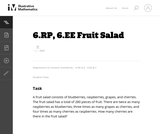Conditions of Use: Remix and Share Rating This is a task from the Illustrative Mathematics website that is one ... This is a task from the Illustrative Mathematics website that is one part of a complete illustration of the standard to which it is aligned. Each task has at least one solution and some commentary that addresses important asects of the task and its potential use. Here are the first few lines of the commentary for this task: A fruit salad consists of blueberries, raspberries, grapes, and cherries. The fruit salad has a total of 280 pieces of fruit. There are twice as many r... Subject: Mathematics Material Type: Activity/Lab Provider: Illustrative Mathematics Provider Set: Illustrative Mathematics Author: Illustrative Mathematics Date created 11-محرم-1436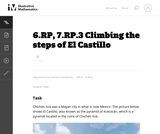Conditions of Use: Remix and Share Rating This is a task from the Illustrative Mathematics website that is one ... This is a task from the Illustrative Mathematics website that is one part of a complete illustration of the standard to which it is aligned. Each task has at least one solution and some commentary that addresses important asects of the task and its potential use. Here are the first few lines of the commentary for this task: Chichén Itzá was a Mayan city in what is now Mexico. The picture below shows El Castillo, also known as the pyramid of Kukulcán, which is a pyramid loc... Subject: Mathematics Material Type: Activity/Lab Provider: Illustrative Mathematics Provider Set: Illustrative Mathematics Author: Illustrative Mathematics Date created 11-محرم-1436Conditions of Use: Remix and Share Rating This is a task from the Illustrative Mathematics website that is one ... This is a task from the Illustrative Mathematics website that is one part of a complete illustration of the standard to which it is aligned. Each task has at least one solution and some commentary that addresses important asects of the task and its potential use. Here are the first few lines of the commentary for this task: Hippos sometimes get to eat pumpkins as a special treat. If 3 hippos eat 5 pumpkins, how many pumpkins per hippo is that? Lindy made 24 jelly-bread san... Subject: Mathematics Material Type: Activity/Lab Provider: Illustrative Mathematics Provider Set: Illustrative Mathematics Author: Illustrative Mathematics Date created 11-محرم-1436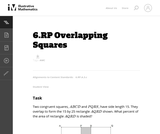Conditions of Use: Remix and Share Rating This is a task from the Illustrative Mathematics website that is one ... This is a task from the Illustrative Mathematics website that is one part of a complete illustration of the standard to which it is aligned. Each task has at least one solution and some commentary that addresses important asects of the task and its potential use. Here are the first few lines of the commentary for this task: Two congruent squares,ABCD$and$PQRS$, have side length 15. They overlap to form the 15 by 25 rectangle$AQRD\$ shown. What percent of the area of re...

Subject:
Mathematics
Material Type:
Activity/Lab
Provider:
Illustrative Mathematics
Provider Set:
Illustrative Mathematics
Author:
Illustrative Mathematics
Date created
11-محرم-1436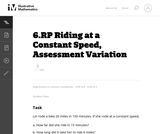Conditions of Use:
Remix and Share
Rating

This is a task from the Illustrative Mathematics website that is one ...

This is a task from the Illustrative Mathematics website that is one part of a complete illustration of the standard to which it is aligned. Each task has at least one solution and some commentary that addresses important asects of the task and its potential use. Here are the first few lines of the commentary for this task: Lin rode a bike 20 miles in 150 minutes. If she rode at a constant speed, How far did she ride in 15 minutes? How long did it take her to ride 6 miles?...

Subject:
Mathematics
Material Type:
Activity/Lab
Provider:
Illustrative Mathematics
Provider Set:
Illustrative Mathematics
Author:
Illustrative Mathematics
Date created
11-محرم-1436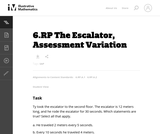Conditions of Use:
Remix and Share
Rating

This is a task from the Illustrative Mathematics website that is one ...

This is a task from the Illustrative Mathematics website that is one part of a complete illustration of the standard to which it is aligned. Each task has at least one solution and some commentary that addresses important asects of the task and its potential use. Here are the first few lines of the commentary for this task: Ty took the escalator to the second floor. The escalator is 12 meters long, and he rode the escalator for 30 seconds. Which statements are true? Select...

Subject:
Mathematics
Material Type:
Activity/Lab
Provider:
Illustrative Mathematics
Provider Set:
Illustrative Mathematics
Author:
Illustrative Mathematics
Date created
11-محرم-1436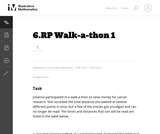Conditions of Use:
Remix and Share
Rating

This is a task from the Illustrative Mathematics website that is one ...

This is a task from the Illustrative Mathematics website that is one part of a complete illustration of the standard to which it is aligned. Each task has at least one solution and some commentary that addresses important asects of the task and its potential use. Here are the first few lines of the commentary for this task: Julianna participated in a walk-a-thon to raise money for cancer research. She recorded the total distance she walked at several different points in ti...

Subject:
Mathematics
Material Type:
Activity/Lab
Provider:
Illustrative Mathematics
Provider Set:
Illustrative Mathematics
Author:
Illustrative Mathematics
Date created
11-محرم-1436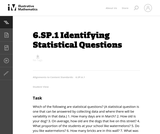Conditions of Use:
Remix and Share
Rating

This is a task from the Illustrative Mathematics website that is one ...

This is a task from the Illustrative Mathematics website that is one part of a complete illustration of the standard to which it is aligned. Each task has at least one solution and some commentary that addresses important asects of the task and its potential use. Here are the first few lines of the commentary for this task: Which of the following are statistical questions? (A statistical question is one that can be answered by collecting data and where there will be variab...

Subject:
Mathematics
Material Type:
Activity/Lab
Provider:
Illustrative Mathematics
Provider Set:
Illustrative Mathematics
Author:
Illustrative Mathematics
Date created
11-محرم-1436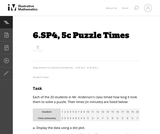Conditions of Use:
Remix and Share
Rating

This is a task from the Illustrative Mathematics website that is one ...

This is a task from the Illustrative Mathematics website that is one part of a complete illustration of the standard to which it is aligned. Each task has at least one solution and some commentary that addresses important asects of the task and its potential use. Here are the first few lines of the commentary for this task: Each of the 20 students in Mr. Anderson's class timed how long it took them to solve a puzzle. Their times (in minutes) are listed below: Student123456...

Subject:
Mathematics
Material Type:
Activity/Lab
Provider:
Illustrative Mathematics
Provider Set:
Illustrative Mathematics
Author:
Illustrative Mathematics
Date created
11-محرم-1436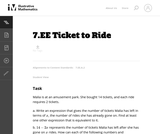Conditions of Use:
Remix and Share
Rating

This is a task from the Illustrative Mathematics website that is one ...

This is a task from the Illustrative Mathematics website that is one part of a complete illustration of the standard to which it is aligned. Each task has at least one solution and some commentary that addresses important asects of the task and its potential use. Here are the first few lines of the commentary for this task: Malia is at an amusement park. She bought 14 tickets, and each ride requires 2 tickets. Write an expression that gives the number of tickets Malia has ...

Subject:
Mathematics
Material Type:
Activity/Lab
Provider:
Illustrative Mathematics
Provider Set:
Illustrative Mathematics
Author:
Illustrative Mathematics
Date created
11-محرم-1436Conditions of Use:
Remix and Share
Rating

This is a task from the Illustrative Mathematics website that is one ...

This is a task from the Illustrative Mathematics website that is one part of a complete illustration of the standard to which it is aligned. Each task has at least one solution and some commentary that addresses important asects of the task and its potential use. Here are the first few lines of the commentary for this task: Below is a table showing the number of hits and the number of times at bat for two Major League Baseball players during two different seasons: SeasonDe...

Subject:
Mathematics
Material Type:
Activity/Lab
Provider:
Illustrative Mathematics
Provider Set:
Illustrative Mathematics
Author:
Illustrative Mathematics
Date created
11-محرم-1436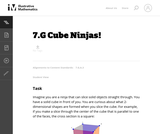Conditions of Use:
Remix and Share
Rating

This is a task from the Illustrative Mathematics website that is one ...

This is a task from the Illustrative Mathematics website that is one part of a complete illustration of the standard to which it is aligned. Each task has at least one solution and some commentary that addresses important asects of the task and its potential use. Here are the first few lines of the commentary for this task: Imagine you are a ninja that can slice solid objects straight through. You have a solid cube in front of you. You are curious about what 2-dimensional ...

Subject:
Mathematics
Material Type:
Activity/Lab
Provider:
Illustrative Mathematics
Provider Set:
Illustrative Mathematics
Author:
Illustrative Mathematics
Date created
11-محرم-1436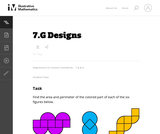Conditions of Use:
Remix and Share
Rating

This is a task from the Illustrative Mathematics website that is one ...

This is a task from the Illustrative Mathematics website that is one part of a complete illustration of the standard to which it is aligned. Each task has at least one solution and some commentary that addresses important asects of the task and its potential use. Here are the first few lines of the commentary for this task: Find the area and perimeter of the colored part of each of the six figures below. The purple, blue, orange, red, and green figures are composed of smal...

Subject:
Mathematics
Material Type:
Activity/Lab
Provider:
Illustrative Mathematics
Provider Set:
Illustrative Mathematics
Author:
Illustrative Mathematics
Date created
11-محرم-1436